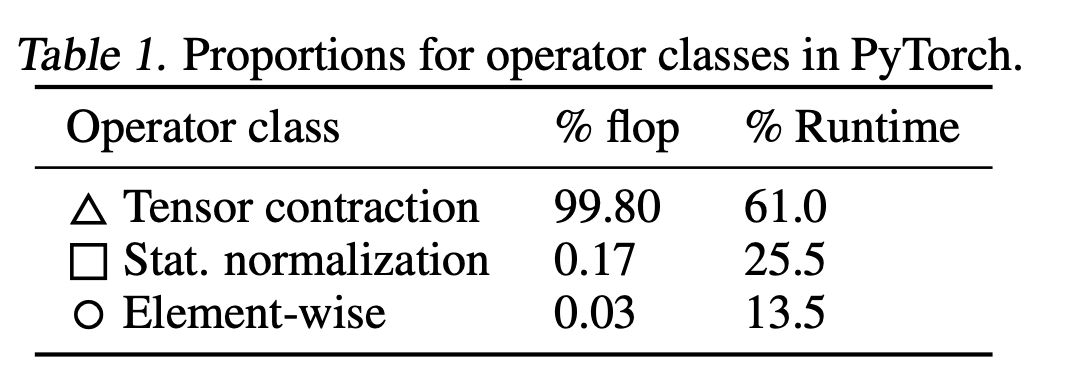# Why is dot product attention faster than additive attention?

In section 3.2.1 of Attention Is All You Need the claim is made that:

Dot-product attention is identical to our algorithm, except for the scaling factor of $$\frac{1}{\sqrt{d_k}}$$. Additive attention computes the compatibility function using a feed-forward network with a single hidden layer. While the two are similar in theoretical complexity, dot-product attention is much faster and more space-efficient in practice, since it can be implemented using highly optimized matrix multiplication code.

It does not make sense why dot product attention would be faster. Additive attention is nearly identical computation wise; the main difference is $$Q + K$$ instead of $$Q K^T$$ in dot product attention. $$Q K^T$$ requires at least as many addition operations as $$Q + K$$, so how can it possibly be faster?

The additive attention method that the researchers are comparing to corresponds to a neural network with 3 layers (it is not actually straight addition). Computing this will involve one multiplication of the input vector by a matrix, then by another matrix, and then the computation of something like a softmax. Smart implementation of a dot-product will not break out the whole matrix multiplication algorithm for it, and it will basically be a tight, easily parallelized loop.

• Do you have a source for the 3 layer structure? If you see the chart comparing attentions here: lilianweng.github.io/lil-log/2018/06/24/… Apr 18, 2019 at 16:41
• Lilian says the only difference between additive and dot product is Q + K vs Q K^T. I don't see any mention of multiple layers to compute Q + K Apr 18, 2019 at 16:41
• @user3180 I think it's right in the quote in your question. " Additive attention computes the compatibility function using a feed-forward network with a single hidden layer. " A single hidden layer means it's a 3 layer network (input + hidden + output) Apr 18, 2019 at 20:21
• That line still doesn't make sense to me. Looking at a reputable implementation of additive attention, they literally just sum the Q + K tensors. Are you implying the network takes in Q concatenated with K, passes through the MLP, to get Q + K? It does not make much sense to call that concatenation + MLP operation as "addition" Apr 18, 2019 at 21:50
• Just think about the parameterizations that lead to Q and K. If they are obtained by some transformations such as Q=f(x; W_Q), K=f(x; W_K), there will be no need to apply additional transformations like Q=f(x; W_Q, A_Q), K=f(x; W_K, A_K). Sep 23, 2020 at 0:46

In additive attention (as described in the paper by Bahdanau et al. (2014)), the alignment model $$a$$ is represented by a feedforward neural network. If you look in their appendix, they actually implement this as

$$e_{ij} = V_a^T \tanh \left(W_a s_{i-1} + U_a h_j\right) = V_a^T \tanh \left(Q + K\right).$$

In contrast, in dot-product attention (as described in the paper by Vaswani et al. (2017)), the alignment model is implemented as

$$e_{ij} = W_Q s_{i-1} \left(W_K h_j\right)^T = Q K^T.$$

The computational advantage is that the dot-product alignment model has only two weight matrices and only needs matrix multiplication, for which highly-optimized code exists.

Two are similar in theoretical complexity.

But matrix multiplication is better optimized. According to this paper, non-matmul ops in transformer are 15x slower than matmul operation.Making Deep Learning Go Brrrr From First Principles discuses about similar topics.